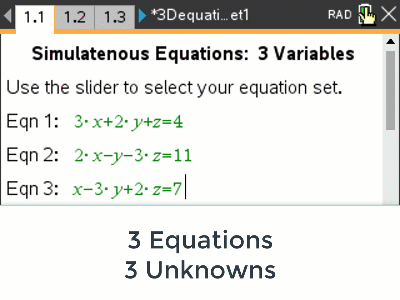# Activities

•• ##### AuthorAust Senior

30 Minutes

• ##### Device
•TI-Nspire™ CX CAS
• ##### Software

TI-Nspire™ CAS

5.0

## 3D Equations#### Activity Overview

Equations of 3 variables can be visualised in three dimensions. In this activity students are provided with 5 sets of three equations. Supporting the understanding and development is a 3D graph for each equation set. Students can see how pairs of graphs interact or all three. An estimate for the point of intersection is gained from the 3D graph. Students are then provided with a scaffolded learning environment and resources where they progressively eliminate a variable. What does it mean graphically when a variable is removed?

#### Objectives

Increase conceptual understanding of solutions to simultaneous equations, specifically those with three variables and including the intermediate steps as variables are successively eliminated.

#### Vocabulary

• Simultaneous Equations
• Substitution
• Elimination
• Linear
• Plane (Flat surface) /